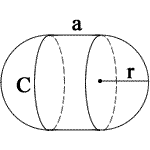## Solid Surface Area calculator

Find surface area for:
 a = r = Units: Surface Area = 0a = side length
» Show Work

### Circular Cone Surface Area

1. Lateral Surface Area = πrs = πr√(r2 + h2)
2. Base Surface Area = πr2
3. Total Surface Area
= L + B = πrs + πr2 = πr(s + r) = πr(r + √(r2 + h2))

### Capsule Surface Area

1. Surface Area = 2πr(2r + a)

### Circular Cylinder Surface Area

1. Top Surface Area = πr2
2. Bottom Surface Area = πr2
3. Total Surface Area
= L + T + B = 2πrh + 2(πr2) = 2πr(h+r)

### Cube Surface Area

1. Surface Area = 6a2

### Conical Frustum Surface Area

1. Lateral Surface Area
= π(r1 + r2)s = π(r1 + r2)√((r1 - r2)2 + h2)
2. Top Surface Area = πr12
3. Base Surface Area = πr22
4. Total Surface Area
= π(r12 + r22 + (r1 * r2) * s)
= π[ r12 + r22 + (r1 * r2) * √((r1 - r2)2 + h2) ]

### Hemisphere Surface Area

1. Curved Surface Area = 2πr2
2. Base Surface Area = πr2
3. Total Surface Area= (2πr2) + (πr2) = 3πr2

### Rectangular Prism Surface Area

1. Surface Area = 2(lw + lh + wh)

### Pyramid Surface Area

1. Lateral Surface Area = a√(a2 + 4h2)
2. Base Surface Area = a2
3. Total Surface Area
= L + B = a2 + a√(a2 + 4h2))
= a(a + √(a2 + 4h2))

### Spherical Cap Surface Area

1. Surface Area = 2πRh

### Sphere Surface Area

1. Surface Area = 4πr2

For example, To sphere when r = 3

1. To calculate the surface area of a sphere, use the following formula: 4πr2. When you substitute r for its value, you get 4π * 32, which equals 113.09734 m2.

For example, To cone when r = 3, h = 3

1. To calculate the surface area of a cone, use the following formula: πr(r + √(r2 + h2)). When you substitute r and h for their values, you get π3(3 + *radic;(32 + 32)), which equals 68.26028 m2.

Thinkcalculator.com provides you helpful and handy calculator resources.